Related Articles

# Surface Tension

• Last Updated : 21 Jul, 2021

Surface tension is the ability of fluid surfaces to contract into the smallest possible surface area. Have you ever found that even after filling a glass full of water, you can only add a few more drops before it spills? Have you ever lost a thermometer and watched how the mercury reacts as it falls? All of which is caused by the surface tension of the surface. Its isolated surface behaves like a strong rubber membrane due to the suppressive force in the fluid molecules. As a result, the individual surface of the fluid is still in a state of stress and tends to have the smallest field. Thus, Surface Tension refers to the tension on the fluid’s individual surface. Let’s discuss more surface tension as:

### Surface Tension

Surface tension is described as the phenomenon that occurs when the surface of a liquid comes into contact with another phase (it can be a liquid as well). Liquids appear to have the smallest possible surface area. The liquid’s surface looks like an elastic sheet.

Imagine a line XY (as shown in the figure below) on the independent surface of the fluid in the equilibrium, then at every point on this line, the same force acts in its exact opposite direction. Every point stretched with the same force in both directions.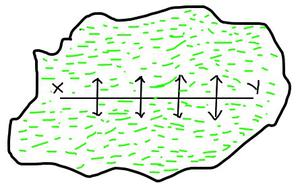Thus, in the equilibrium, a force acting on any other per unit length of an imaginary line on an independent surface of the fluid, which is perpendicular to the line and in the direction of the tangent line of the surface, is called Surface Tension

Surface tension is denoted by σ or T symbol.

Mathematically, the surface tension is defined as the force (F) acting on the surface and the length (l) of the surface, so is given as:

T = F / l

Also, the ratio of the work done (W) and the change in the area of the surface (A) is termed as the surface tension.

T = W / A

Its SI unit is N/m or J/m and its dimensional formula is [M L0 T-2].

### Cause of the Surface Tension

The effect known as surface tension is caused by the cohesive forces between liquid molecules. Since the molecules at the surface lack like molecules in both directions, they cohere more closely to those specifically aligned with them on the surface. This creates a surface “film” that makes moving an object across the surface more difficult than moving it while fully submerged.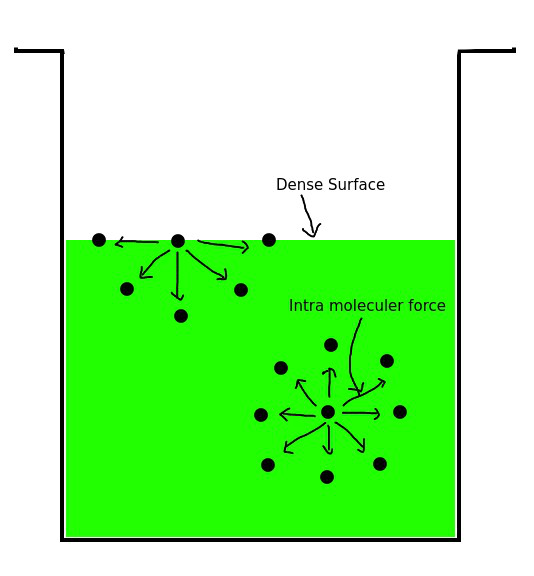Assume a jar is filled with water; the water molecules can be found in two positions in this jar: First, beneath the water, and second, on the surface of the water. Since there are no molecules above these molecules, the molecules at the water’s surface are unbalanced. As a result, only the molecules below will be attracted. As a result, a thin crust will form on the liquid’s upper surface. Because of this thick layer, a form of stress is generated, which is known as Surface Tension. These phenomena can also be explained in terms of energy.

### Surface Energy

Surface energy measures the breakdown of intermolecular bonds caused by the formation of a surface. Surface free energy and interfacial free energy are other names for it. Surface energy is defined as the work done per unit area by the force that forms the new surface.×

When the free surface area of a liquid is increased, effort must be done against the force of surface tension. This work is stored as potential energy on the liquid surface. This increased potential energy per unit area of free surface of liquid is referred to as surface energy. Mathematically, the surface energy is defined as:

Surface energy = Surface tension × Change in the surface area

or

ES = T × ΔA

where T denotes surface tension and ΔA denotes an increase in surface area.

Therefore, the SI unit of surface energy is Nm-2 and dimensional formula is [MT– 2].

### Angle of Contact

The angle of contact is defined as the angle subtended between the tangents drawn at the liquid surface and the solid surface within the liquid at the point of contact, or it is defined as the angle subtended between the tangents drawn at the liquid surface and the solid surface within the liquid at the angle of contact (θ).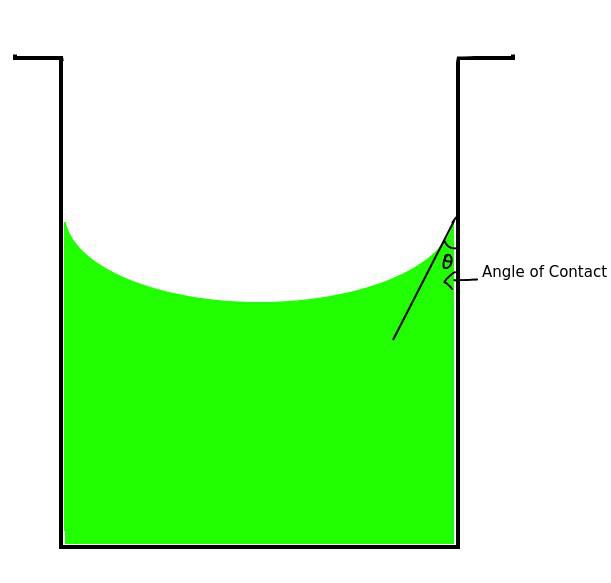The angle of contact depends on the following factors:

• The nature of the liquid, the solid with which it comes into contact.
• The medium that exists above the free surface of the liquid.
• As the temperature of the liquid rises, so does the angle of contact.
• When soluble impurities are added to a liquid, the angle of contact drops.

### Tendency of Fluids to Reduce the Surface Area

Imagine a drop of water, which is spherical as shown below. There are two types of molecules that appears in the drop of water. First, those who are situated on the surface of a drop of water. Second those who are situated under the drop of water.

• The molecule of fluid located in the droplet which is located inside the droplet will be attracted by the molecules around it due to sensory force. These molecules of fluid will have some amount of negative potential energy, because some energy required to separate this molecule of fluid from this drop of fluid.
• Let’s assume that this molecule of fluid has X negative potential energy. Now consider the molecule which is located on the free surface of the droplet.  Because this molecule is on the surface of the droplet, therefore this molecule will not be surrounded by the fluid molecules from around it. Due to which the value of sensory force applied on it will be half as compared to the first molecule.
• In this case, the negative potential energy of this molecule will also be half the value, which will be X/2, which required less energy to separate this molecule from a drop of fluid. According to this, we can say that the energy level of the molecules on the surface is high, due to which they try to bring more and more molecules to lower energy levels and this happens until the minimum possible energy remains on the surface of the fluid to maintain the surface. This is why fluids try to cover the least amount of space.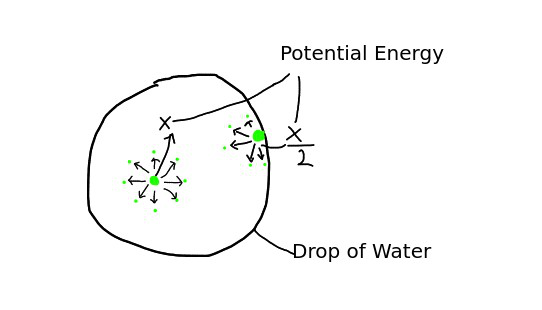### Applications of Surface Tension

1. The spherical shape of droplets: Small droplets of fluid are spherical due to surface tension. The molecules of water tend to stick together due to intramolecular force, and the energy of molecules which are located on the surface of droplets contain higher energy and try to push the other molecule to the center of the droplet. Due to this the drop makes the shape which contains the least surface area and the spherical shape is best for the least surface area, that’s why The droplets of water and raindrops are spherical.
2. Fire polishing of glass: The method of polishing glass or thermoplastic with the help of fire or flame is called Fire Polishing. When we heat a glass material in flames, the glass surface starts melting. But due to surface tension, it starts to become soft and smooth which makes the glass very flat and smooth. This method is most applicable to flat external surfaces. Flame polishing is frequently in acrylic plastic fabrication because of its high speed compared to abrasive methods. In this application, an oxyhydrogen torch is typically used, one reason being that the flame chemistry is unlikely to contaminate the plastic.
3. The rise of liquids in Capillary Tubes: A tube whose radius is very short and uniform are called a capillary tube. When an open capillary is dipped in water. The water rises to some height in the capillary tube.

Suppose there is a bowl of water in which we dip the capillary tube, then according to figure we can see that there are two points on the surface of the fluid. One that is independent and one that is inside the tube. We see that the atmospheric pressure at A and B is P, but the pressure of fluid P0 at point B will be less than atmospheric pressure P. due to which the molecules of the fluid will go to the low-pressure part to balance the pressure, due to which the water in the capillary tube goes up as in figure 2.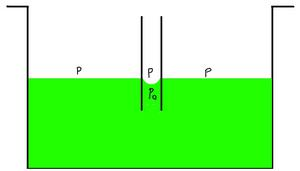Figure 1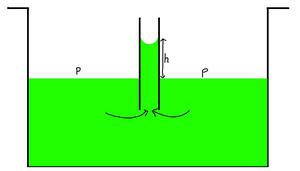Figure 2

The difference between two pressures is, P – P0 = 2T/R

Let’s assume the height of rises water is h then the equation will be as follows:

h = 2T cos θ / rρg

where T is the surface tension, θ is the angle of contact, ρ is the radius of the capillary tube, r is the density of water and g is the gravitational acceleration.

### Factors affecting Surface Tension

• If the solute is highly soluble in the fluid, the surface tension of the fluid would increase. And if the solute is less soluble in the fluid, then the surface tension of the fluid would decrease.
• If there are dust particles or any lubricant present on the surface of the fluid, the surface tension of the fluid decreases.
• Increasing the temperature reduces the surface tension of the fluid. And decreasing the temperature increases the surface tension.

### Sample Problems

Problem 1: Why soap is helpful in cleaning clothes?

Solution:

Soap reduces the surface tension of water.  So water penetrates into small areas of clothing and removes stains. So soap is helpful for washing clothes.

Problem 2: Why do we use Toothpaste to clean teeth?

Solution:

Toothpaste froth reduces surface tension. Hence, it clears the teeth by failing over a large area of teeth.

Problem 3: Why are small drops of mercury flat and big drops flattened?

Solution:

There are two types of forces acting on mercury droplets. On small droplets, the surface tension is higher than the gravitational force, so it is rounded but on big droplets, the surface tension is lower than gravitational force, so it is flattened.

Problem 4: Why do clothes get cleaned faster in warm water instead of cold water?

Solution:

On heating the water, its surface tension is reduces, due to which it spreads clothes in more area than cold water and cleans clothes.

Problem 5: Why do we use Dettol to wash wounds?

Solution:

The surface tension of water is reduced due to which is penetrates the small cracks of the wound and cleans the wound.

Attention reader! All those who say programming isn’t for kids, just haven’t met the right mentors yet. Join the  Demo Class for First Step to Coding Course, specifically designed for students of class 8 to 12.

The students will get to learn more about the world of programming in these free classes which will definitely help them in making a wise career choice in the future.

My Personal Notes arrow_drop_up